# box

Display axes outline

## Syntax

``box on``
``box off``
``box``
``box(ax,___)``

## Description

example

````box on` displays the box outline around the current axes by setting their `Box` property to `'on'`. This property value is the default for `GeographicAxes` objects.```
````box off` removes the box outline around the current axes by setting their `Box` property to `'off'`. This property value is the default for `Axes` and `PolarAxes` objects.```
``box` toggles the display of the box outline.`

example

````box(ax,___)` uses the axes specified by `ax` instead of the current axes. Specify the axes as the first input argument for any of the previous syntaxes. Use single quotes around the `'on'` and `'off'` inputs, such as `box(ax,'on')`.```

## Examples

collapse all

Plot a surface and display the box outline around the axes.

```[X,Y,Z] = peaks; surf(X,Y,Z) box on```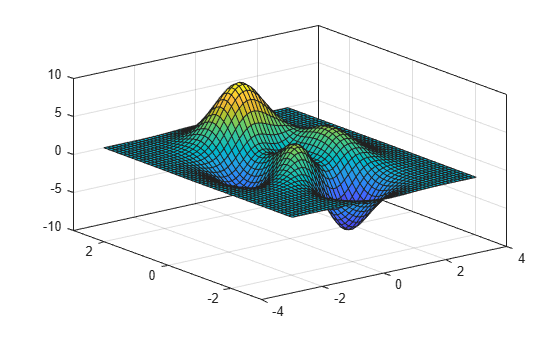Turn off the display of the box outline.

`box off`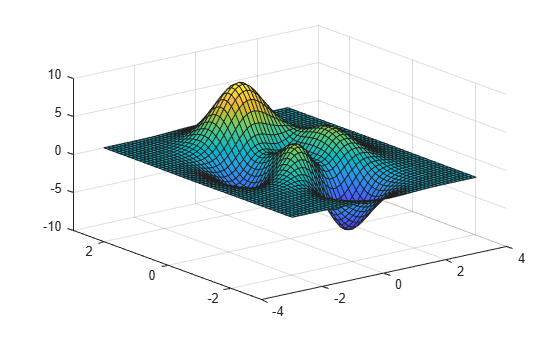First, plot a surface and display the box outline around the axes. By default, the outline appears around the back planes of the axes because the `BoxStyle` property of the axes is set to `'back'`.

```[X,Y,Z] = peaks; surf(X,Y,Z) box on```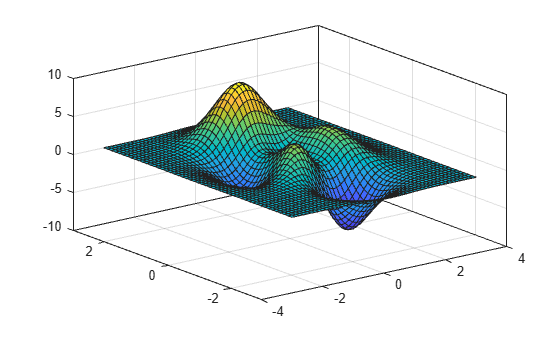Next, display the outline around the entire axes by setting the `BoxStyle` property to `'full'`. Use dot notation to set properties.

```ax = gca; ax.BoxStyle = 'full';```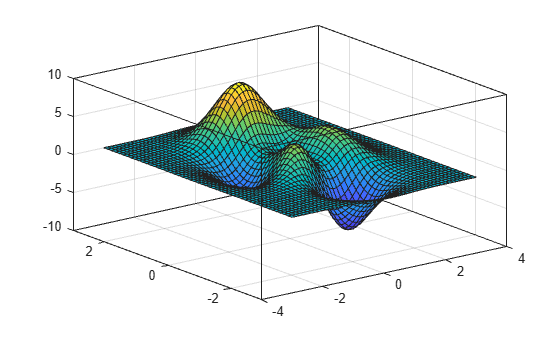Create a scatter plot and display the box outline around the axes.

```x = rand(10,1); y = rand(10,1); scatter(x,y) box on```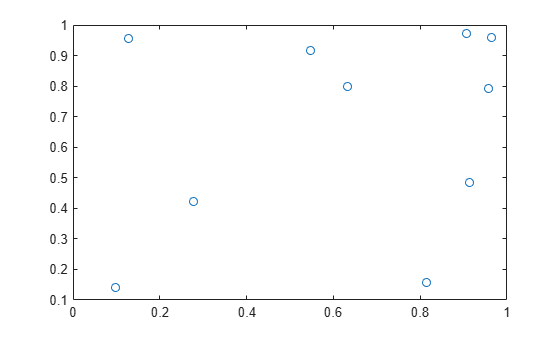Change the color of the box outline in the x-axis direction by setting the `XColor` property of the axes. Use dot notation to set properties.

```ax = gca; ax.XColor = 'red';```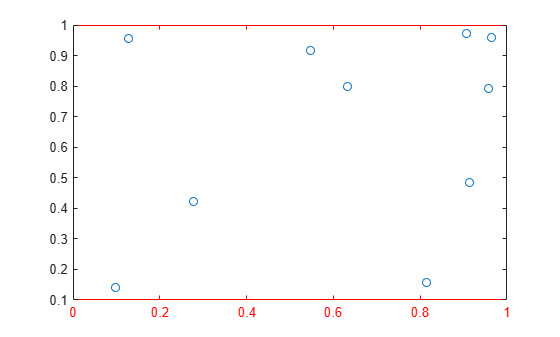Create two axes using the `tiledlayout` and `nexttile` functions. Assign the axes objects to the variables `ax1` and `ax2`, and plot into the axes. Remove the box outline around the lower plot by specifying `ax2` as the first input argument to `box`.

```tiledlayout(2,1) ax1 = nexttile; plot(ax1,1:10) ax2 = nexttile; plot(ax2,1:10) box(ax2,'off')```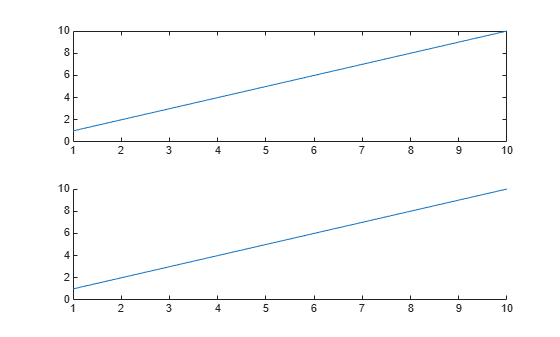## Input Arguments

collapse all

Target axes, specified as one of the following:

• Any type of axes object: an `Axes`, `PolarAxes`, or `GeographicAxes` object.

• An array of axes objects that belong to the same class. To determine the class, use the `class` function.

If you do not specify the axes, then `box` modifies the current axes.

## Algorithms

The `box` function sets the `Box` property of the `Axes`, `PolarAxes`, or `GeographicAxes` object to either `'on'` or `'off'`.